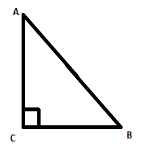Courses
Courses for Kids
Free study material
Free LIVE classes
MoreLIVE
Join Vedantu’s FREE Mastercalss

# If $\angle A$ and $\angle B$ are acute angles such that $\cos A=\cos B$ , then show that$\angle A=\angle B$.Verified
270.9k+ views
Hint: Use the fact that $\cos A=\dfrac{adjacent}{hypotenuse}$
Then apply the condition $\cos A=\operatorname{cosB}$

Complete step by step solution:
Let us first take a right angled triangle $ABC$ .It is given that $\angle A$ and $\angle B$ are acute angles. Acute angle are those whose measure lies
between ${{0}^{\circ }}$ to ${{90}^{\circ }}$ . In the figure we can clearly see that $\angle A$ and $\angle B$ are bigger than ${{0}^{\circ }}$ and smaller than ${{90}^{\circ }}$ .
So these two angles are acute angles.
$\angle C$ is a right angle. That means $\angle C={{90}^{\circ }}$ .
Therefore triangle $ABC$ is a right angled triangle.
We know that in a right angled triangle, the cosine of an angle is:
The length of the adjacent side is divided by the length of the hypotenuse.
In a right angled triangle, the hypotenuse is the longest side and the opposite side of the right angle. An adjacent side is the side which is next to a given angle.
So, here the opposite side of the right angle is $AB$ . Hence $AB$ is the hypotenuse.
Now, for $\angle A$ the adjacent side or the side which is next to $\angle A$ is $AC$ .

Therefore, $\cos A=\dfrac{adjacent}{hypotenuse}=\dfrac{AC}{AB}$
Similarly, for $\angle B$ the adjacent side or the side which is next to $\angle B$ is $CB$ .
Therefore, $\cos B=\dfrac{CB}{AB}$
Now in the question it is given that $\operatorname{cosA}=\cos B$
\begin{align} & \cos A=\cos B \\ & \Rightarrow \dfrac{AC}{AB}=\dfrac{CB}{AB} \\ \end{align}
We can cancel out $AB$ from both sides, as both are in the denominator. So, now we have:
$\Rightarrow AC=CB$
Therefore, in triangle $ABC$ two sides are equal.
That makes the triangle $ABC$ an isosceles triangle.
We know that, in isosceles triangle the two angles opposite to the equal sides are congruent to each
another or we can say the opposite angles are equal to each other.
The two angles opposite to the equal sides are $\angle A$ and $\angle B$ .
Therefore, $\angle A=\angle B$
Hence, we proved that, if $\angle A$ and $\angle B$ are acute angles such that $\cos A=\cos B$ , then
$\angle A=\angle B$.

Note: Here we have to take the correct ratio for $\cos A$ , otherwise we will get the wrong answer. We
generally get confused with other trigonometric ratios. So be careful about that.
Last updated date: 27th Sep 2023
Total views: 270.9k
Views today: 5.70k